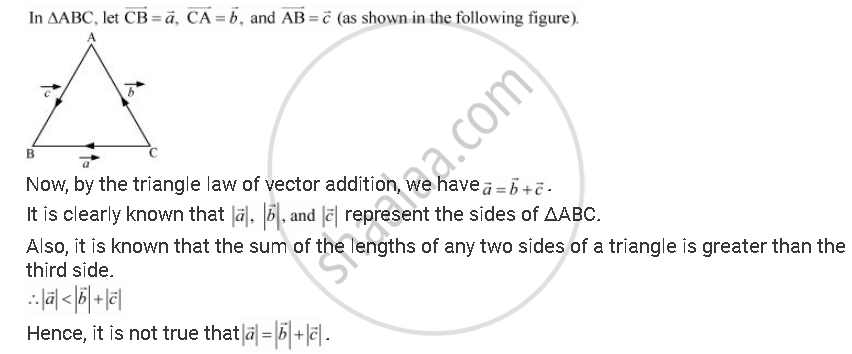Share

# If Veca = Vecb + Vecc, Then is It True that |Veca| = |Vecb| + |Vecc|? Justify Your Answer. - CBSE (Commerce) Class 12 - Mathematics

#### Question

If veca = vecb + vecc, then is it true that |veca| = |vecb| + |vecc|? Justify your answer.

#### SolutionIs there an error in this question or solution?

#### Video TutorialsVIEW ALL 

Solution If Veca = Vecb + Vecc, Then is It True that |Veca| = |Vecb| + |Vecc|? Justify Your Answer. Concept: Addition of Vectors.
S